# PengRobinson EOS in FPROPS

Work on this is went on as a part of GSoC 2010 (Project Ankit) and continues as part of GSoC 2011 (Richard Towers). See also FPROPS.

## Overview

The Peng-Robinson EOS is a cubic equation of state in that it contains volume terms to the third power. It is usually expressed to give pressure in terms of temperature and molar volume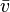${\bar v}$: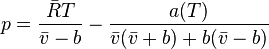$p =\frac{{\bar R} T}{{\bar v}-b}-\frac{a(T)}{{\bar v}({\bar v}+b)+b({\bar v}-b)}$

where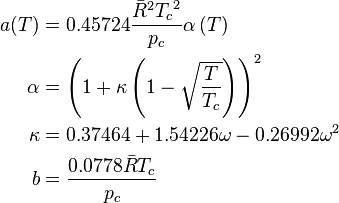\begin{align} a(T) &= 0.45724 \frac{{\bar R}^2{T_c}^2}{p_c} \alpha \left(T \right) \\ \alpha &= \left( 1+\kappa \left( 1-\sqrt{\frac{T}{T_c}} \right) \right)^2 \\ \kappa &= 0.37464+1.54226\omega - 0.26992\omega^2 \\ b &= \frac{0.0778\bar R T_c}{p_c} \end{align}

It is sometimes more convenient to express the equation as a cubic polynomial in terms of compressibility factor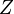$Z$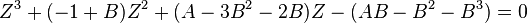$Z^3+(-1+B)Z^2+(A-3B^2-2B)Z-(AB-B^2-B^3)=0$

in which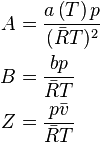\begin{align} A &= \frac{a \left(T \right) p}{({\bar R} T)^2} \\ B &= \frac{b p}{{\bar R} T} \\ Z &= \frac{p {\bar v}}{{\bar R} T} \end{align}

## Departure Functions

Departure functions represent the departure of the real properties from the ideal properties - i.e the properties of a fluid at zero pressure or infinite molar volume. The departure functions of the Peng-Robinson equation of state are as follows: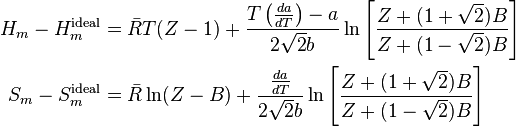\begin{align} H_{m}-H_{m}^{\text{ideal}}&={\bar R} T(Z-1)+\frac{T\left(\frac{da}{dT}\right)-a}{2\sqrt{2}b}\ln\left[\frac{Z+(1+\sqrt{2})B}{Z+(1-\sqrt{2})B}\right] \\ S_{m}-S_{m}^{\text{ideal}}&={\bar R} \ln (Z-B)+\frac{\frac{da}{dT}}{2\sqrt{2}b}\ln\left[\frac{Z+(1+\sqrt{2})B}{Z+(1-\sqrt{2})B}\right] \end{align}

Clearly to evaluate these functions we need to be able to evaluate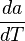$\frac{da}{dT}$ (checked, agrees with Sandler):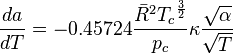$\frac{da}{dT}= -0.45724 \frac{{\bar R}^{2} {T_c}^{\frac{3}{2}} }{p_c} \kappa \frac{\sqrt{\alpha} }{ \sqrt{T}}$